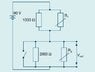# Output Voltage

TyErd

## Homework Statement

The circuit below combines variable resistors R1 and R2 with fixed resistors to make a complex voltage divider.

If the switch is closed and R1=8000ohms and R2 is 5000ohms, what is the output voltage.

V=IR

## The Attempt at a Solution

the equivalent resistor of 1000 and 8000 is 3077 and the current through that is 0.03A. I am not sure what to do after that.

#### Attachments

•circuit1.JPG
6.2 KB · Views: 328

Staff Emeritus
Gold Member
You do not need to know the resistor values. Look at the diagram and think about it.

TyErd
um..a current goes through the first parallel part and then splits up when entering the next parallel part...

TyErd
not sure whrre to look

TyErd
...?

TyErd
is it 0 because the current will go through the part of the circuit where there's the least resistance?

willem2
is it 0 because the current will go through the part of the circuit where there's the least resistance?

Yes. All the current will go through the closed switch and none of it through the two resistances that are parallel with it.
The output voltage is I_switch * R_switch and R_switch = 0.

Although it's not relevant for the answer, the equivalent resistance of 1000 ohm and
8000 ohm resistances in parallel is not 3077 ohm. The equivalent resistance of 2 parallel resistances should be smaller then the smallest of the resistances.

TyErd
okay thnx, my mistake the equivalent resistor should be 889ohms. Question, why does the current only go through the switch? Is it because its the easiest path?